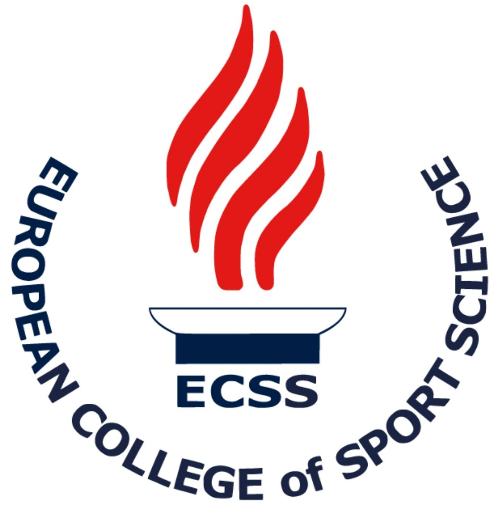Sassi A1, Rampinini E1, Impellizzeri FM1

1,Human Performance Lab, Sport Service MAPEI, Castellanza, ItalyThe distances covered in selected speed zones measured by video match-analysis systems are commonly used to evaluate the physical work of soccer players during match-play. However, the use of speed ranges to quantify the effort may underestimate the physiological strain because it does not take into account the short sprints and striding, where the player does not reach speed values classified as high intense activities. The aim of this study was to compare the quantification of the high intense match activities using the classification based on running speed ranges, to that determined from the estimation of the energy cost of running including acceleration and decelerations. Match speed raw data of 10 players during official matches (Serie A) were determined using a commercial video-computerized, semi-automatic, match analysis system (SICS, Bassano del Grappa, Italy). Three intensity zones based on speed ranges were determined: walking (0-2.2 m/s), running (2.2-4.4 m/s), and sprinting (>4.4 m/s). For the same intensity zones, the speed thresholds were converted in metabolic power: 0-5.4 W /kg for walking, 5.4-16.2 W/kg for running, and >16.2 W/kg for sprinting. The movement energy cost including speed changes was calculated adding, to the cost of walking (2.5 J/kg/m) or running (3.6 J/kg/m), the energy spent for kinetic energy variations. The latter was calculated assuming a player’s body mass of 75 kg, and an efficiency of 25% for the mechanical work performed while accelerating, and four times higher for the negative work performed while decelerating. Using the speed ranges method, the distances covered while walking, running and sprinting were 4094±454 m, 5067±1182 m and 1585±381 m, respectively. These values corresponded to 38%, 47% and 15% of the total distance covered (10745±1072 m), respectively. Using the metabolic power thresholds the distance covered while walking and running decreased to 2721±355 m and 4094±723 m (P<0.001) corresponding to  25% and 38% of total distance, respectively. The distance covered sprinting increased to 3930±672 m (P<0.001) or 37% of total distance. During soccer match, the total energy cost estimated from the average distance covered at the various intensities was 29.6±3.6 kJ/kg. If speed changes were taken into account the energy cost increased by 77%, i.e. 52.4±5.4 kJ/kg (P>0.001). Our results confirmed that classifying the physical strain using the speed categories method the physiological effort is underestimated. In fact, taking into account the frequent acceleration and deceleration running phases, the distance covered at high metabolic cost increased from 15 to 37%.  However, the total energy cost estimated with the present method is still lower than the about 70 kJ/kg reported in the literature, suggesting that the total energy cost is influenced by other factors such as the additional cost of unorthodox movements and running with the ball, or a higher cost of running on grass surface.

10th Annual Congress of the European College of Sport Science in Lausanne, Switzerland 2006.